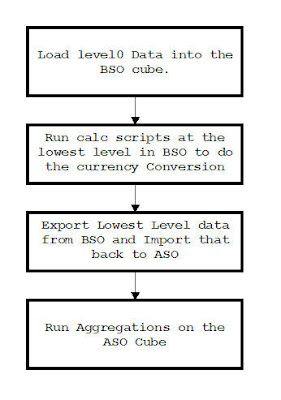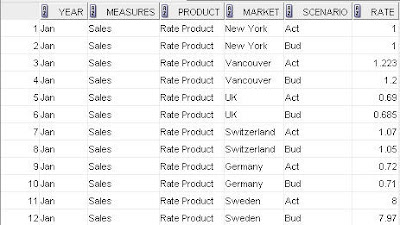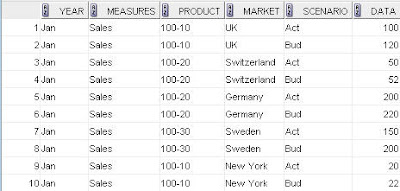# Archive for January 5th, 2009

## Hyperion Essbase 9.3.1 – Currency Conversion in ASO Cubes – Using Level0 Export from BSO & ASO Aggregation – Approach 2

Posted by Venkatakrishnan J on January 5, 2009

In the previous blog entry here, i had covered an approach to do Currency Conversion in ASO Cubes. In most cases, that would suffice unless the currency rate dimension has more than 1000 or more dependent members. In such cases where are a lot of interdependent members, we can use another approach wherein the conversions happen in the BSO cube but ASO is used for reporting and aggregation. The basic idea behind this approach is provided belowThe above approach works only if you have data coming in at the lowest level in your BSO cube. If there is any data at the top levels(either as an user input in Excel Add-in or through the data load), then those values would be ignored during the import into ASO.To understand this better, we shall use the same ASO & BSO outlines which we built out in the articles here and here. To recap, our BSO outline is same as the outline in Sample->Interntl database. The only addition is the new member called “Rate Product” in the Product dimension to accomodate rate loads.The assumption is that we would be loading local currency data only at the lowest level. We would be using the same rule files that we built on the blog entries above to load the data and rates. To give a perspective on the input data and rates, their corresponding screenshots are provided hereNow use the below calculation script to convert the local currency to USD.

```SET CACHE HIGH;
SET CALCPARALLEL 2;

FIX("Sales",@LEVMBRS("Market",0))
"Actual" = "Act" / "Act"->"Rate Product";
"Budget" = "Bud" / "Bud"->"Rate Product";
"Actual @ Bud XChg" = "Act" / "Bud"->"Rate Product";
ENDFIX;
```

The above calculation script will basically convert the local currency to USD numbers. And the USD numbers would be stored under the Actual and Budget members. Once this is done, the next step is to take a level0 export of the BSO cube using the below MaxL script

```export database 'Sample'.'Interntl' level0 data to data_file 'D:\BSOExport.exp';
```

The above step completes the first phase of the currency coversion. In the second phase, we would have to prepare the ASO outline in such a way that the import of data from the BSO cube happens correctly. We shall start with the same ASO outline that we migrated from BSO in the approach 1. There are a couple of changes which are needed on this ASO outline. They are

1. Remove the formula from the Actual, Budget and Act @ XChg Budget members.
2. Convert the dynamic hierarchies to stored hierarchies in the Scenario dimension

The converted ASO outline is shown belowOnce the ASO outline is ready, the next step is to import the exported level0 BSO data. This is achieved through the maxL script shown below

```import database 'CurrASO'.'CurrASO' data from data_file 'D:\BSOExport.exp' on error abort;
```

Once the data is loaded inside the ASO cube, aggregations need to be performed. Once the aggregations are done, lets verify the results in HVE.The entire process described above can be automated through a combination of batch(or shell scripts in Unix) and maxL scripts. Of course, the scripts given above are not final and binding as there can be multiple variations depending on the requirements. But this should give an idea of how to go about doing currency conversions in ASO as well as BSO cubes.